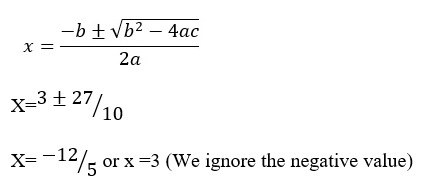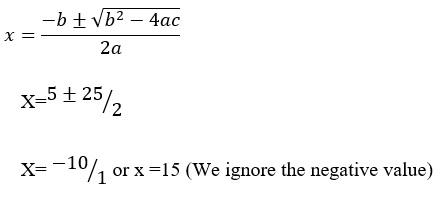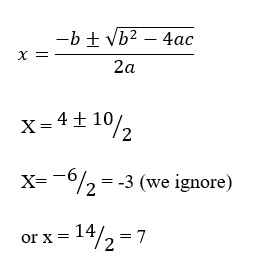# Linear Equations Sample Assignment

1. An integer is 3 less than 5 times another. If the product of the two integers is 36, then find the integers.

Let one of the integer be x

The other integer will be 5x-3

Therefore; x(5x-3) = 36

5x2-3x-36=0Therefore, x=3

Hence the integers are 3 and 5(3)-3=12

2) The width of a rectangle is 5 units less than the length. If the area is 150 square units, then find the dimensions of the rectangle.

Let the length of the rectangle be x units

Width will be x-5 Units

X(x-5) = 150

5x2-3x-36=0Therefore, x = 15

Hence; The dimensions are;

Length = x units = 15 units

Width = x-5 = 15-5 = 10units

• The length of a rectangle is 4 inches more than its width. The area of the rectangle is equal to 5 inches more than 2 times the perimeter. Find the length and width of the rectangle.

Let the width = x

Length = x+4

Area = 3xPerimeter+5

X(x+4)=2(2(l+b)+5)

X2+4x=4(2x+4)+5

x2-4x-21=0Therefore, length = x+4 = 7+4 = 11 inches

Width = x = 7 Inches

• The height of a projectile launched upward at a speed of 32 feet/second from a height of 128 feet is given by the function ℎ(𝑡)=−16𝑡2+32𝑡+128. How long will it take the projectile to hit the ground?

Let h = 0, since height of the ground is zero.

−16𝑡2+32𝑡+128=0

-16(t+2)(t-4)=0

t = -2 or t = 4

Time cannot be a negative value so we ignore t = -2

Therefore, the projectile will hit the ground in 4 Seconds.

• The height of an object dropped from the top of a 144-foot building is given by ℎ(𝑡) = −16𝑡2+144. How long will it take the object to hit the ground?

The ball will hit the ground at h=0

−16𝑡2+144 = 0

T2=9

t = 3 Seconds

Therefore; The ball will hit the ground in 3 Seconds.